# Calculate source count rates and fluxes## Overview

#### Synopsis:

CIAO provides a number of tools for calculating count rates and source fluxes - e.g. aprates, modelflux, eff2evt - as described in the Compute Net Counts, Rate, or Flux for Point Sources thread. The srcflux script simplifies the running of these tools.

Please note that srcflux can only be used with Chandra imaging mode data.

you want to calculate net counts, source rate, photon flux, and/or energy flux for ACIS or HRC data, and do not need to follow the manual steps described in Compute Net Counts, Rate, or Flux for Point Sources.

Last Update: 23 Feb 2022 - Review for CIAO 4.14. Updated for Repro5/CALDB 4.9.6.

## Get Started

`unix% download_chandra_obsid 6436 `

For this thread we are going to reprocess the data and use fluximage to create an image of the ACIS-I array. This is shown in case you wish to follow along, but are not required steps for using srcflux!

### Reprocessing the data

Here we reprocess the data, using chandra_repro, to make sure we are using the latest calibration products. This step may not need to be taken for your dataset.

```unix% cd 6436
unix% punlearn chandra_repro
unix% chandra_repro mode=h
```

### A quick look at the data

The fluximage script is used to create a fluxed image of the ACIS-I array, as shown in Figure 1. For this example we use a bin size of 4, so each pixel in the output image is 1.968 arcsecond on a side, which is significantly larger than the on-axis PSF. For your own analysis you may wish to use a smaller binsize value.

```unix% punlearn fluximage
unix% fluximage "repro/acisf06436_repro_evt2.fits[ccd_id=0:3]" fimg/ bands=broad,csc bin=4
Running fluximage
Version: 04 November 2021

Using CSC ACIS broad science energy band.
Using CSC ACIS soft science energy band.
Using CSC ACIS medium science energy band.
Using CSC ACIS hard science energy band.

The output images will have 566 by 565 pixels, pixel size of 1.968 arcsec,
and cover x=2916.5:5180.5:4,y=3012.5:5272.5:4.

Running tasks in parallel with 4 processors.
Creating 4 aspect histograms for obsid 6436
Creating 16 instrument maps for obsid 6436
Creating 16 exposure maps for obsid 6436
Combining 4 exposure maps for 4 bands (obsid 6436)
Thresholding data for obsid 6436
Exposure-correcting 4 images for obsid 6436
Creating 4 PSF maps for obsid 6436

The following files were created:

The clipped counts images are:
fimg/soft_thresh.img
fimg/medium_thresh.img
fimg/hard_thresh.img

The observation FOV is:
fimg/6436.fov

The clipped exposure maps are:
fimg/soft_thresh.expmap
fimg/medium_thresh.expmap
fimg/hard_thresh.expmap

The PSF maps are:
fimg/soft_thresh.psfmap
fimg/medium_thresh.psfmap
fimg/hard_thresh.psfmap

The exposure-corrected images are:
fimg/soft_flux.img
fimg/medium_flux.img
fimg/hard_flux.img
```

### Figure 1: Three-color view of the field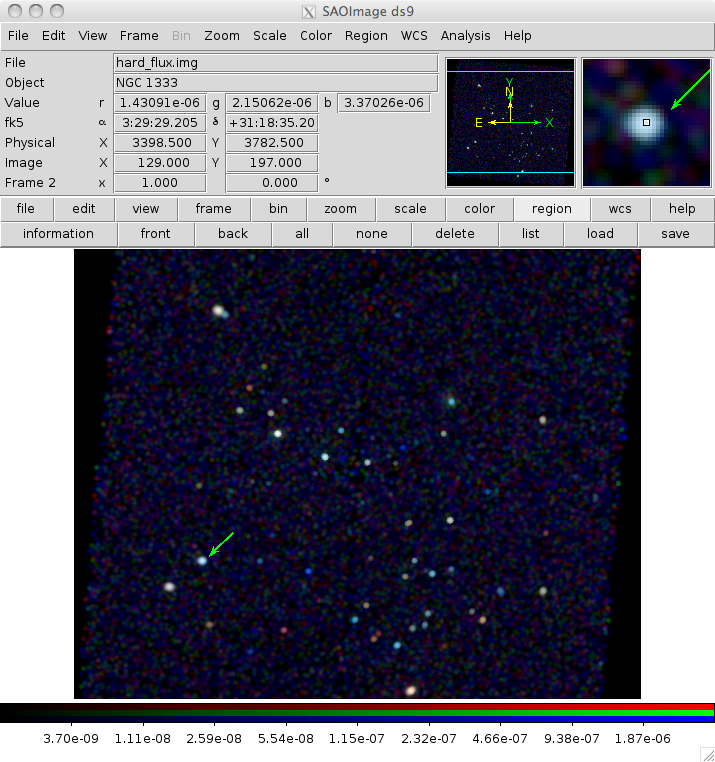### Figure 1: Three-color view of the field

```unix% cd fimg
unix% ds9 -rgb -red soft_flux.img -green medium_flux.img -blue hard_flux.img \
-rgb lock scale yes -rgb lock smooth yes -log -smooth
```

The field contains a number of sources, with varying brightness and X-ray spectra, since there are a range of colors in this image. The background appears relatively flat, and the source density is not really high, which simplifies the calculation of source and background apertures later in the thread.

The arrow indicates the source used in the A single source section.

As of CIAO 4.11 fluximage now automatically creates the PSF map at the same energies used to create the exposure maps for each energy band when the psfecf parameter is set. The broad band psfmap is shown in Figure 2.

### Figure 2: PSF variation across the field of view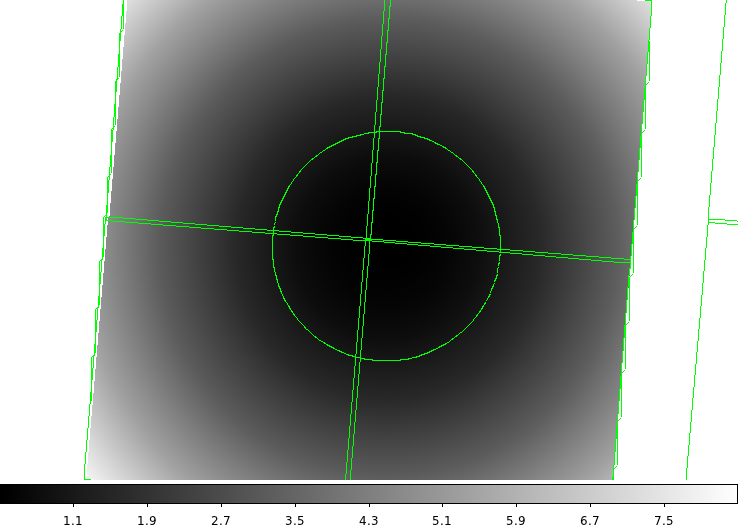### Figure 2: PSF variation across the field of view

The image was created by saying

```unix% dmlist broad_thresh.psfmap header,clean,raw | grep BUNIT
BUNIT        = arcsec               / Units after BSCALE applied
unix% ds9 broad_thresh.psfmap -region ../repro/acisf06436_000N002_fov1.fits \
-contour yes -contour levels "1 "
```

so that the circular region shows the location where the PSF increases to 1 arcsecond (the BUNIT keyword lists the units of the image), and the boxes show the outlines of the ACIS chips (using the FOV files).

## A single source

We shall start by finding the flux of one source; later sections will show how to run with multiple sources.

For this run we shall use the source close to 3400,3800 (Figure 1). As srcflux needs the celestial location, we use dmstat to get a more accurate location and then feed it through dmcoords to do the conversion (you can read the value dircetly from ds9 or use any other technique to get the location).

```unix% dmstat "fimg/broad_flux.img[sky=circle(3423,3795,20)]" cen+
PFLUX_IMAGE(x, y)[/cm**2 /s]
min:	0 	      @:	( 3414.5 3778.5 )
max:	3.2662243029e-05 	      @:	( 3422.5 3794.5 )
cntrd[log] :	( 6.3121935222 6.351405338 )
cntrd[phys]:	( 3423.7487741 3795.9056214 )
sigma_cntrd:	( 0.0097475444081 0.0097340885214 )
good:	77
null:	44
unix% dmcoords fimg/broad_flux.img opt=sky x=3423.75 y=3795.91
unix% set ra = `pget dmcoords ra`
unix% set dec = `pget dmcoords dec`
unix% echo "\$ra \$dec"
03:29:29.252 +31:18:34.62
```

We are now ready to run srcflux (the unimaginative output name is to make it easier to compare with later runs of srcflux):

```unix% punlearn srcflux
unix% srcflux repro/acisf06436_repro_evt2.fits "\$ra \$dec" single/run1
srcflux
infile = repro/acisf06436_repro_evt2.fits
pos = 03:29:29.252 +31:18:34.62
outroot = single/run1
bands = default
srcreg =
bkgreg =
bkgresp = yes
psfmethod = ideal
psffile =
conf = 0.9
binsize = 1
rmffile =
arffile =
model = xspowerlaw.pow1
paramvals = pow1.PhoIndex=2.0
absmodel = xsphabs.abs1
absparams = abs1.nH=%GAL%
abund = angr
fovfile =
asolfile =
mskfile =
bpixfile =
dtffile =
ecffile = CALDB
parallel = yes
nproc = INDEF
tmpdir = ./
random_seed = -1
clobber = yes
verbose = 1
mode = ql

Processing OBI 001
Extracting counts
Setting Ideal PSF : alpha=1 , beta=0
Getting net rate and confidence limits
Getting model independent fluxes
Getting model fluxes
Getting photon fluxes
Running tasks in parallel with 4 processors.
Making response files for single/run1_0001
Running modeflux for region 1
Using GAL=0.1464 for source 1
Appending flux results onto output
Appending photflux results onto output
Computing Net fluxes
Scaling model flux confidence limits

Summary of source fluxes

Position                               0.5 - 7.0 keV
Value        90% Conf Interval
#0001|3 29 29.25 +31 18 34.6  Rate           0.0392 c/s (0.0375,0.0409)
Flux           4.98E-13 erg/cm2/s (4.76E-13,5.19E-13)
Mod.Flux       4.38E-13 erg/cm2/s (4.19E-13,4.57E-13)
Unabs Mod.Flux 5.38E-13 erg/cm2/s (5.15E-13,5.62E-13)
```

The count rate and flux estimates are displayed on the screen and written to the output file, which here is single/run1_broad.flux.

```unix% dmlist single/run1_broad.flux"[cols net_rate_aper,net_flux_aper,net_mflux_aper,net_umflux_aper]" data,clean
#  NET_RATE_APER        NET_FLUX_APER        NET_MFLUX_APER       NET_UMFLUX_APER
0.03917760      4.975567703E-13      4.379663904E-13      5.380651584E-13
```

The errors are also included; for instance the model-independent flux estimate:

```unix% dmlist single/run1_broad.flux"[cols net_flux_aper,net_flux_aper_lo,net_flux_aper_hi]" data,clean
#  NET_FLUX_APER        NET_FLUX_APER_LO     NET_FLUX_APER_HI
4.975567703E-13      4.759146459E-13       5.19330975E-13
```

Since we did not supply any region information (the srcreg and bkgresp parameters were left empty), the script calculated a source aperture radius based on the 90% enclosed-count fraction of the PSF at 1 keV for the source location, and the background is an annulus around this. The output file contains information on the source and aperture regions (there are also ROTANG and COMPONENT columns), as shown below.

```unix% dmlist single/run1_broad.flux"[cols shape,r,area,bg_area]" data,clean
#  SHAPE              R                                     AREA                 BG_AREA
Circle                        9.624140                    0              290.250             6981.750
```

For this particular source, which is not on-axis (it is 6 arc minutes off axis), the size of the point source is significantly larger than the on-axis performance, which is why the radius used is 5 arcseconds.

```unix% dmlist single/run1_broad.flux"[cols theta,phi]" data,clean
#  THETA                PHI
6.0420333736       298.7815830774

--------------------------------------------------------------------------------
Columns for Table Block HISTOGRAM
--------------------------------------------------------------------------------

ColNo  Name                 Unit        Type             Range
1   THETA                arcmin       Real8          -Inf:+Inf            Off axis angle
2   PHI                  deg          Real8          -Inf:+Inf            Azimuth angle
```

Since psfmethod=ideal, the count rate and fluxes are not corrected to account for the finite PSF size. This can be seen by looking at the PSFFRAC and BG_PSFFRAC columns, which give the enclosed-count fraction (ECF) of the PSF for the source and background aperture repectively:

```unix% dmlist single/run1_broad.flux"[cols psffrac,bg_psffrac]" data,clean
#  PSFFRAC              BG_PSFFRAC
1.0                    0
```

The output-file section describes the columns in the output file.

In this example we left the various *file parameters of srcflux empty, which means that it used the header keywords in the event file to find them: if this does not work, or you want to use your own versions of these files, then set the appropriate parameter - aspect solution, mask, bad pixel, and the FOV file - before running the script. If these files cannot be located some of the flux values cannot be computed and will be returned as NAN.

The script also creates spectra and responses, so that you can analyze them yourself, if they have enough counts.

```unix% ls -1 single/run1_*
single/run1_0001.arf
single/run1_0001.pi
single/run1_0001.rmf
single/run1_0001_6436.fov
single/run1_0001_bkg.pi
single/run1_0001_bkgreg.fits
single/run1_0001_grp.pi
single/run1_0001_nopsf.arf
single/run1_0001_reg.fits
single/run1_0001_srcreg.fits
single/run1_summary.txt

unix% sherpa

>>> subtract()
>>> group_counts(20)
>>> ignore(None, 0.6)
>>> ignore(7,None)
>>> set_source(xsphabs.abs1 * xspowerlaw.pow1)
>>> abs1.nh = 0
>>> pow1.phoindex = 2
>>> calc_energy_flux(0.5, 7)
4.2305760894875184e-09
>>> f0 = calc_energy_flux(0.5, 7)
>>> pow1.norm.val *= 4.384556677E-13 / f0
>>> calc_energy_flux(0.5, 7)
4.3845566770000115e-13
>>> plot_fit()
>>> import matplotlib.pylab as plt
>>> plt.xscale("log")
>>> plt.yscale("log")
```

which creates Figure 3.

### Figure 3: Spectrum of the source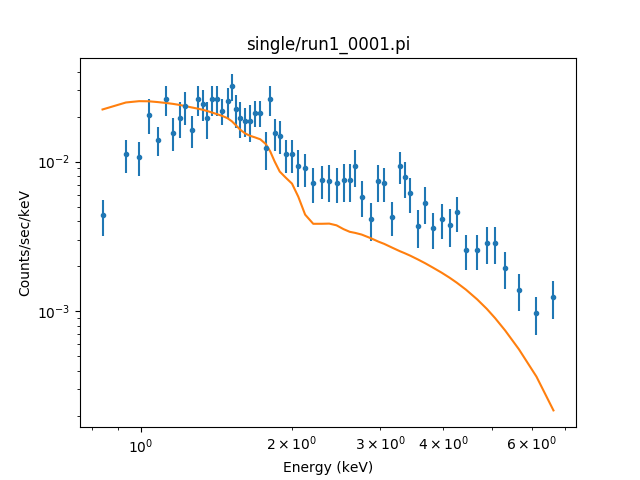### Figure 3: Spectrum of the source

Since the analysis was performed on a bright source there are enough counts to fit a model and calculate the flux from that, for this source. Here we include, as a red line, the model that srcfluc used to calculate the NET_MFLUX_APER value; line 11 adjusts the normalization of the model so that the flux is the same as calculated by the script. As can be seen, for this source the model is not a good fit, so the parameter values need adjusting or a more appropriate model expression should be used.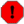PSF correction and the ARF created by srcflux

The ARFs saved by srcflux for each source - such as single/run1_0001.arf in the example above - have been corrected for aperture size (i.e. by running arfcorr on them) - whatever the psfmethod parameter setting is.

### Correcting for Galactic absorption

We are going to change the absorbing column value using prop_colden, as shown below:

```unix% prop_colden d nrao eval 3 29 29.25 31 18 34.62
Looking for /export/miniconda/envs/ciao-4.14/config/jcm_data/nrao.fits
------------------------------------------------------------------------------------------
Input coords:  03 29 29.25 +31 18 34.62
Target RA,Dec (B1950): 03 26 23.597 +31 08 17.471   (l,b):158.397679   -20.466884
Density integrated from   -550.000 to    550.000 km/s
Hydrogen density (10^20 cm**(-2)):  14.64
------------------------------------------------------------------------------------------
```

With this value we change the model used so that the absorbing component is moved to the absmodel parameter, remembering to convert from the units of 1020 cm-2 provided by prop_colden to 1022 cm-2 required by the xsphabs model:

```unix% pset srcflux model=xspowerlaw.pow1 paramvals="pow1.phoindex=2"
unix% pset srcflux absmodel=xsphabs.abs1 absparams="abs1.nh=0.1464"
unix% srcflux repro/acisf06436_repro_evt2.fits "\$ra \$dec" single/run2
srcflux
infile = repro/acisf06436_repro_evt2.fits
pos = 03:29:29.252 +31:18:34.62
outroot = single/run2
bands = default
srcreg =
bkgreg =
bkgresp = yes
psfmethod = ideal
psffile =
conf = 0.9
binsize = 1
rmffile =
arffile =
model = xspowerlaw.pow1
paramvals = pow1.phoindex=2
absmodel = xsphabs.abs1
absparams = abs1.nh=0.1464
abund = angr
fovfile =
asolfile =
mskfile =
bpixfile =
dtffile =
ecffile = CALDB
parallel = yes
nproc = INDEF
tmpdir = ./
random_seed = -1
clobber = yes
verbose = 1
mode = ql

Processing OBI 001
Extracting counts
Setting Ideal PSF : alpha=1 , beta=0
Getting net rate and confidence limits
Getting model independent fluxes
Getting model fluxes
Getting photon fluxes
Running tasks in parallel with 4 processors.
Making response files for single/run2_0001
Running modeflux for region 1
Appending flux results onto output
Appending photflux results onto output
Computing Net fluxes
Scaling model flux confidence limits

Summary of source fluxes

Position                               0.5 - 7.0 keV
Value        90% Conf Interval
#0001|3 29 29.25 +31 18 34.6  Rate           0.0392 c/s (0.0375,0.0409)
Flux           4.98E-13 erg/cm2/s (4.76E-13,5.19E-13)
Mod.Flux       4.38E-13 erg/cm2/s (4.19E-13,4.57E-13)
Unabs Mod.Flux 5.38E-13 erg/cm2/s (5.15E-13,5.62E-13)
```

So, in comparison to the original run, the model flux and unabsorbed model flux values are the same. This is because the original run used the special token %GAL% which the tool automatically replaces with the galactic nH value. The NET_RATE_APER and NET_FLUX_APER values do not change, since their values are model independent):

```unix% dmlist single/run\*_broad.flux"[cols net_rate_aper,net_flux_aper,net_mflux_aper,net_umflux_aper]" data,clean
#  NET_RATE_APER        NET_FLUX_APER        NET_MFLUX_APER       NET_UMFLUX_APER
0.03917760      4.975567703E-13      4.379663904E-13      5.380651584E-13
#  NET_RATE_APER        NET_FLUX_APER        NET_MFLUX_APER       NET_UMFLUX_APER
0.03917760      4.975567703E-13      4.379663904E-13      5.380651584E-13
```Changing the model

Although we changed the absorbing model parameter, we did not change the source model from the default value of a power law with a photon index of 2. This should be changed to match the expected properties of the source, or sources, being analyzed.

### PSF correction

If we set psfmethod=quick, then an aperture correction will be made to the count rate and fluxes:

```unix% srcflux repro/acisf06436_repro_evt2.fits "\$ra \$dec" single/run3 psfmethod=quick
srcflux
infile = repro/acisf06436_repro_evt2.fits
pos = 03:29:29.252 +31:18:34.62
outroot = single/run3
bands = default
srcreg =
bkgreg =
bkgresp = yes
psfmethod = quick
psffile =
conf = 0.9
binsize = 1
rmffile =
arffile =
model = xspowerlaw.pow1
paramvals = pow1.phoindex=2
absmodel = xsphabs.abs1
absparams = abs1.nh=0.1464
abund = angr
fovfile =
asolfile =
mskfile =
bpixfile =
dtffile =
ecffile = CALDB
parallel = yes
nproc = INDEF
tmpdir = ./
random_seed = -1
clobber = yes
verbose = 1
mode = ql

Processing OBI 001
Extracting counts
Getting net rate and confidence limits
Getting model independent fluxes
Getting model fluxes
Getting photon fluxes
Running tasks in parallel with 4 processors.
Making response files for single/run3_0001
Running modeflux for region 1
Appending flux results onto output
Appending photflux results onto output
Computing Net fluxes
Scaling model flux confidence limits

Summary of source fluxes

Position                               0.5 - 7.0 keV
Value        90% Conf Interval
#0001|3 29 29.25 +31 18 34.6  Rate           0.0451 c/s (0.0431,0.0471)
Flux           5.72E-13 erg/cm2/s (5.48E-13,5.98E-13)
Mod.Flux       5.04E-13 erg/cm2/s (4.82E-13,5.26E-13)
Unabs Mod.Flux 6.19E-13 erg/cm2/s (5.92E-13,6.46E-13)
```

The same regions are used as before, but this time the PSF fraction is calculated, and used to correct the count rate and flux estimates (the fraction of the PSF that falls in the background area is assumed to be zero):

```unix% dmlist "single/run3_broad.flux[cols shape,r,area,bg_area]" data,clean
#  SHAPE              R                                     AREA                 BG_AREA
Circle                        9.624520                    0              290.250            6982.1250
#  PSFFRAC              BG_PSFFRAC
0.86911376973698                    0
#  NET_RATE_APER        NET_FLUX_APER        NET_MFLUX_APER       NET_UMFLUX_APER
0.03917760      4.975567703E-13      4.379663904E-13      5.380651584E-13
#  NET_RATE_APER        NET_FLUX_APER        NET_MFLUX_APER       NET_UMFLUX_APER
0.03917760      4.975567703E-13      4.379663904E-13      5.380651584E-13
#  NET_RATE_APER        NET_FLUX_APER        NET_MFLUX_APER       NET_UMFLUX_APER
0.04507770      5.724875012E-13      5.039236083E-13      6.190971318E-13
```

The dmlist output shows the results for the three runs; the last run has larger values, by 1/0.8692 = 1.150.

There are three other settings for the psfmethod parameter:

arfcorr

A model of the PSF is used to calculate the enclosed-count fraction (ECF) for both the source and background apertures (the background region is assumed to be free of source counts for both the ideal and quick options). This model is generated by the arfcorr tool.

Repeating the analysis, but with psfmethod=arfcorr, returns (using outroot=single/run4):

```unix% dmlist "single/run4_broad.flux[cols psffrac,bg_psffrac]" data,clean
#  PSFFRAC              BG_PSFFRAC
0.86415357249724     0.11565451978130
#  NET_RATE_APER        NET_FLUX_APER        NET_MFLUX_APER       NET_UMFLUX_APER
0.04559010      5.789950228E-13      5.096517279E-13      6.261344334E-13
```

The count rate and fluxes are slightly higher than the psfmethod=quick (run3) results because of the fact that BG_PSFFRAC is no longer zero.

psffile

This supports providing an image of the PSF, rather than using the model of arfcorr. Users can run ChaRT and MARX to generate an image of the PSF with the specific spectral model of the source.

marx

Users can also choose marx, which will run marx to simulate the PSF for each source automatically.

### Specifying the region

So far the source and background region has been calculated for us by srcflux, but you can also specify them, as shown in this section. Further examples are discussed in the multiple-source section.

For this example we shall use the following manually-defined regions (no attempt has been made to ensure that the background region is free of contamination by other sources):

Source
```unix% cat src.reg
circle(3423,3795,20)
```
Background
```unix% cat bg.reg
annulus(3423,3795,30,60)
```

We run it with psfmethod=arfcorr to see how good our choice of the background region is. Note that we have not changed the pos parameter, which corresponds in this case to a slightly different location than the center of the source region (since we used rounded values in src.reg and bg.reg). The value given in the pos parameter is used to define the off-axis angle for the PSF corrections.

```unix% srcflux repro/acisf06436_repro_evt2.fits "\$ra \$dec" single/run5 src=src.reg bkgreg=bg.reg psfmethod=arfcorr
srcflux
infile = repro/acisf06436_repro_evt2.fits
pos = 03:29:29.252 +31:18:34.62
outroot = single/run5
bands = default
srcreg = src.reg
bkgreg = bg.reg
bkgresp = yes
psfmethod = arfcorr
psffile =
conf = 0.9
binsize = 1
rmffile =
arffile =
model = xspowerlaw.pow1
paramvals = pow1.phoindex=2
absmodel = xsphabs.abs1
absparams = abs1.nh=0.1464
abund = angr
fovfile =
asolfile =
mskfile =
bpixfile =
dtffile =
ecffile = CALDB
parallel = yes
nproc = INDEF
tmpdir = ./
random_seed = -1
clobber = yes
verbose = 1
mode = ql

Processing OBI 001
Extracting counts
Making PSF models
Getting PSF fraction by running arfcorr 1
Combining PSF fractions together
Getting net rate and confidence limits
Getting model independent fluxes
Getting model fluxes
Getting photon fluxes
Running tasks in parallel with 4 processors.
Making response files for single/run5_0001
Running modeflux for region 1
Appending flux results onto output
Appending photflux results onto output
Computing Net fluxes
Scaling model flux confidence limits

Summary of source fluxes

Position                               0.5 - 7.0 keV
Value        90% Conf Interval
#0001|3 29 29.25 +31 18 34.6  Rate           0.0432 c/s (0.0414,0.045)
Flux           5.59E-13 erg/cm2/s (5.36E-13,5.83E-13)
Mod.Flux       4.85E-13 erg/cm2/s (4.65E-13,5.05E-13)
Unabs Mod.Flux 5.96E-13 erg/cm2/s (5.71E-13,6.21E-13)
```

There is now a small contamination of the background by the PSF:

```unix% dmlist single/run5_broad.flux"[cols psffrac,bg_psffrac]" data,clean
#  PSFFRAC              BG_PSFFRAC
0.97200144088498     0.00303585880048
```

### Upper limits

Here we have picked a location with no obvious source emission (Figure 4). When given to srcflux, upper limits are returned

```unix% punlearn srcflux
unix% srcflux repro/acisf06436_repro_evt2.fits "3:29:01.24 +31:19:30.72" ulim/src
srcflux
infile = repro/acisf06436_repro_evt2.fits
pos = 3:29:01.24 +31:19:30.72
outroot = ulim/src
bands = default
srcreg =
bkgreg =
bkgresp = yes
psfmethod = ideal
psffile =
conf = 0.9
binsize = 1
rmffile =
arffile =
model = xspowerlaw.pow1
paramvals = pow1.PhoIndex=2.0
absmodel = xsphabs.abs1
absparams = abs1.nH=%GAL%
abund = angr
fovfile =
asolfile =
mskfile =
bpixfile =
dtffile =
ecffile = CALDB
parallel = yes
nproc = INDEF
tmpdir = ./
random_seed = -1
clobber = yes
verbose = 1
mode = ql

Processing OBI 001
Extracting counts
Setting Ideal PSF : alpha=1 , beta=0
Getting net rate and confidence limits
Getting model independent fluxes
Getting model fluxes
Getting photon fluxes
Running tasks in parallel with 4 processors.
Making response files for ulim/src_0001
Running modeflux for region 1
Using GAL=0.1474 for source 1
Appending flux results onto output
Appending photflux results onto output
Computing Net fluxes
Scaling model flux confidence limits

Summary of source fluxes

Position                               0.5 - 7.0 keV
Value        90% Conf Interval
#0001|3 29 1.24 +31 19 30.7   Rate           0 c/s (NAN,6.31E-05)
Flux           NAN erg/cm2/s (NAN,NAN)
Mod.Flux       0 erg/cm2/s (NAN,6.22E-16)
Unabs Mod.Flux 0 erg/cm2/s (NAN,7.64E-16)
```

The best estimates are either 0 or NaN, with upper limits given in the _HI fields:

```unix% dmlist ulim/src_broad.flux"[cols upper_limit]" data,clean
#  UPPER_LIMIT
TRUE
#  NET_RATE_APER        NET_FLUX_APER        NET_MFLUX_APER
0                  NaN                    0
#  NET_RATE_APER_HI     NET_FLUX_APER_HI     NET_MFLUX_APER_HI
6.31156E-05                  NaN      6.215624288E-16
```

### Figure 4: An area with no obvious source emission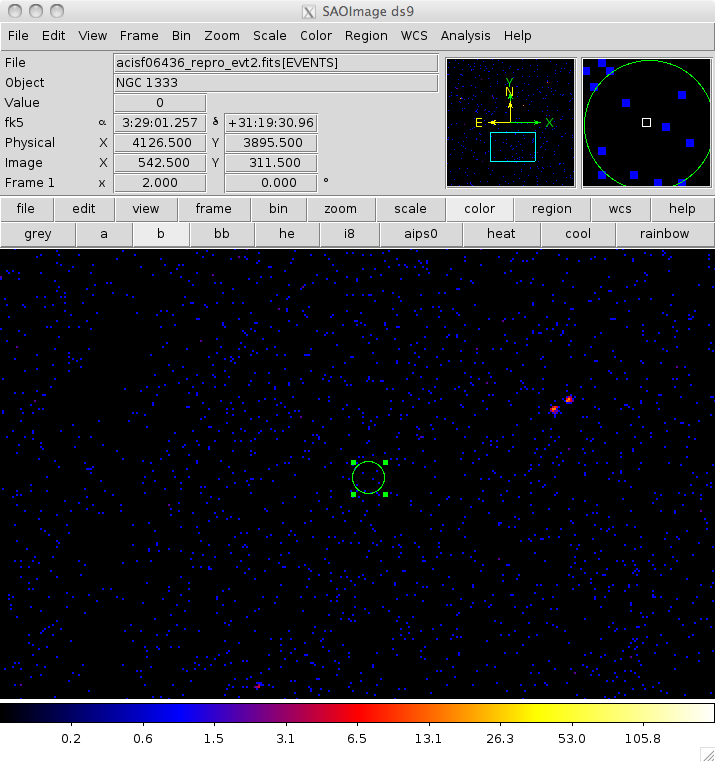### Figure 4: An area with no obvious source emission

The circle indicates the location of a region with no obvious source emission.Regions with no counts

If the region contains no counts - e.g. because the location is close to the aim point of the observation and so the extraction radius used by srcflux is small - then an error from fluximage will be reported and the upper limits will not be calculated, but will be set to NaN.

### Off chip

In this section we show two examples that show what can happen when the source lies outside of, or overlaps, the field of view of the observation.

We start with a source that lies far enough outside the FOV that the source aperture does not cover the observation at all:

```unix% punlearn srcflux
unix% srcflux repro/acisf06436_repro_evt2.fits "3:29:48 +31:27:20" offchip/run1
srcflux
infile = repro/acisf06436_repro_evt2.fits
pos = 3:29:48 +31:27:20
outroot = offchip/run1
bands = default
srcreg =
bkgreg =
bkgresp = yes
psfmethod = ideal
psffile =
conf = 0.9
binsize = 1
rmffile =
arffile =
model = xspowerlaw.pow1
paramvals = pow1.PhoIndex=2.0
absmodel = xsphabs.abs1
absparams = abs1.nH=%GAL%
abund = angr
fovfile =
asolfile =
mskfile =
bpixfile =
dtffile =
ecffile = CALDB
parallel = yes
nproc = INDEF
tmpdir = ./
random_seed = -1
clobber = yes
verbose = 1
mode = ql

Processing OBI 001
Extracting counts
Setting Ideal PSF : alpha=1 , beta=0
Getting net rate and confidence limits
Getting model independent fluxes
Getting model fluxes
Getting photon fluxes
Running tasks in parallel with 4 processors.
Making response files for offchip/run1_0001
Appending flux results onto output
Appending photflux results onto output
Computing Net fluxes
Scaling model flux confidence limits

Summary of source fluxes

Position                               0.5 - 7.0 keV
Value        90% Conf Interval
#0001|3 29 48.00 +31 27 20.0  Rate           NAN c/s (NAN,NAN)
Outside FOV                  Flux           NAN erg/cm2/s (NAN,NAN)
Mod.Flux       NAN erg/cm2/s (NAN,NAN)
Unabs Mod.Flux NAN erg/cm2/s (NAN,NAN)
```

The Outside FOV screen warning indicates that the source position has been determined to lie outside the field-of-view (FOV) of the observation. As there is no overlap, the count rate and fluxes are all set to NaN.

In the output file, the source is flagged as lying outside the field of view by its INSIDE_FOV value, and the count-rate and flux measurements are set to NaN. The region stored in the output is no longer a Circle, but a Point at (0,0), because the source region lies outside the FOV area for the observation: this is why the source aperture AREA is 0, but the background aperture BG_AREA is non-zero since it partly overlaps the observation in this case.

```unix% dmlist "offchip/run1_broad.flux[cols inside_fov,upper_limit,net_rate_aper,net_rate_aper_lo,net_rate_aper_hi]" data,clean
#  INSIDE_FOV UPPER_LIMIT NET_RATE_APER        NET_RATE_APER_LO     NET_RATE_APER_HI
FALSE  FALSE                  NaN                  NaN                  NaN
#  SHAPE              sky(X,Y)                                 R                                     AREA                 BG_AREA
Point                                 0                    0                    0                    0                    0            3887.8750
```

We now pick a position that is just inside the FOV but near enough to the chip edge that the source and background apertures extend outside it:

```unix% srcflux repro/acisf06436_repro_evt2.fits "3:29:43.5 +31:26:35" overlap/run1
srcflux
infile = repro/acisf06436_repro_evt2.fits
pos = 3:29:43.5 +31:26:35
outroot = overlap/run1
bands = default
srcreg =
bkgreg =
bkgresp = yes
psfmethod = ideal
psffile =
conf = 0.9
binsize = 1
rmffile =
arffile =
model = xspowerlaw.pow1
paramvals = pow1.PhoIndex=2.0
absmodel = xsphabs.abs1
absparams = abs1.nH=%GAL%
abund = angr
fovfile =
asolfile =
mskfile =
bpixfile =
dtffile =
ecffile = CALDB
parallel = yes
nproc = INDEF
tmpdir = ./
random_seed = -1
clobber = yes
verbose = 1
mode = ql

Processing OBI 001
Extracting counts
Setting Ideal PSF : alpha=1 , beta=0
Getting net rate and confidence limits
Getting model independent fluxes
Getting model fluxes
Getting photon fluxes
Running tasks in parallel with 4 processors.
Making response files for overlap/run1_0001
Running modeflux for region 1
Using GAL=0.1481 for source 1
Appending flux results onto output
Appending photflux results onto output
Computing Net fluxes
Scaling model flux confidence limits

Summary of source fluxes

Position                               0.5 - 7.0 keV
Value        90% Conf Interval
#0001|3 29 43.49 +31 26 35.0  Rate           0 c/s (NAN,0.000118)
Flux           1.37E-15 erg/cm2/s (NAN,1.37E-15)
Mod.Flux       0 erg/cm2/s (NAN,3.82E-15)
Unabs Mod.Flux 0 erg/cm2/s (NAN,4.7E-15)
```

In this case values were calculated, since the source and background apertures are not zero, but because there is no actual source at this position an upper limit is returned.

```unix% dmlist "overlap/run1_broad.flux[cols shape,x,y,r,area,bg_area]" data,clean
#  SHAPE              sky(X,Y)                                 R                                     AREA                 BG_AREA
Circle                               3054.120      4772.750            23.37850                    0              1352.50          22675.43750
#  INSIDE_FOV UPPER_LIMIT NEAR_CHIP_EDGE
TRUE   TRUE   TRUE
#  NET_RATE_APER        NET_RATE_APER_LO     NET_RATE_APER_HI
0                  NaN          0.000117733
```Note

There is no indication, either on screen, or in the output file - e.g. overlap/run1_broad.flux - that an aperture has been reduced because it extends outside the FOV. The NEAR_CHIP_EDGE column can be used to see if the source lies near a chip edge, which indicates that there may be truncation of the background or source regions.

## The output file

There is one output file per band which contains the source count rate and fluxes. If multiple sources are used then the table will contain multiple rows. Here we show the output of the first run:

```unix% dmlist single/run1_broad.flux blocks

--------------------------------------------------------------------------------
--------------------------------------------------------------------------------

Block Name                          Type         Dimensions
--------------------------------------------------------------------------------
Block    1: PRIMARY                        Null
Block    2: HISTOGRAM                      Table        64 cols x 1        rows
Block    3: GTI3                           Table         2 cols x 1        rows
Block    4: GTI6                           Table         2 cols x 1        rows
Block    5: GTI7                           Table         2 cols x 1        rows
Block    6: GTI2                           Table         2 cols x 1        rows
Block    7: GTI1                           Table         2 cols x 1        rows
Block    8: GTI0                           Table         2 cols x 3        rows
```

The file resembles that created by dmextract when used in its "spatial extraction" mode (e.g. see the Estimate Source Counts in an Event File and Obtain and Fit a Radial Profile threads), but with extra columns added:

```unix% dmlist single/run1_broad.flux cols

--------------------------------------------------------------------------------
Columns for Table Block HISTOGRAM
--------------------------------------------------------------------------------

ColNo  Name                 Unit        Type             Range
1   sky(X,Y)             pixel        Real8          -Inf:+Inf            Position
2   EQPOS(RA,Dec)        deg          Real8          -360.0:      360.0   Position
3   SHAPE                             String                          Region shape type
4   R                 pixel        Real8(2)       -Inf:+Inf            Radius
5   ROTANG            pixel        Real8(2)       -Inf:+Inf            Angle
6   COMPONENT                         Int2           -                    Component number
7   COUNTS               count        Real8          -Inf:+Inf            Counts
8   ERR_COUNTS           count        Real8          -Inf:+Inf            Error on counts
9   AREA                 pixel**2     Real8          -Inf:+Inf            Area of extraction
10   EXPOSURE             s            Real8          -Inf:+Inf            Exposure time of source file
11   COUNT_RATE           count/s      Real8          -Inf:+Inf            Rate
12   COUNT_RATE_ERR       count/s      Real8          -Inf:+Inf            Rate Error
13   BG_COUNTS            count        Real8          -Inf:+Inf            Background Counts
14   BG_ERR               count        Real8          -Inf:+Inf            Error on Background counts
15   BG_AREA              pixel**2     Real8          -Inf:+Inf            Background Area of Extraction
16   BG_EXPOSURE          s            Real8          -Inf:+Inf            Exposure time of background file
17   BG_RATE              count/s      Real8          -Inf:+Inf            Background Rate
18   BG_SUR_BRI           count/pixel**2 Real8          -Inf:+Inf            Background Counts per square pixel
19   BG_SUR_BRI_ERR       count/pixel**2 Real8          -Inf:+Inf            Error on background counts per square pixel
20   NET_COUNTS           count        Real8          -Inf:+Inf            Net Counts
21   NET_ERR              count        Real8          -Inf:+Inf            Error on Net Counts
22   NET_RATE             count/s      Real8          -Inf:+Inf            Net Count Rate
23   ERR_RATE             count/s      Real8          -Inf:+Inf            Error Rate
24   SUR_BRI              count/pixel**2 Real8          -Inf:+Inf            Net Counts per square pixel
25   SUR_BRI_ERR          count/pixel**2 Real8          -Inf:+Inf            Error on net counts per square pixel
26   XPOS                 pixel        Real8          -Inf:+Inf            X center
27   YPOS                 pixel        Real8          -Inf:+Inf            Y center
28   THETA                arcmin       Real8          -Inf:+Inf            Off axis angle
29   PHI                  deg          Real8          -Inf:+Inf            Azimuth angle
30   RAPOS                deg          Real8          -Inf:+Inf            Right Ascencion
31   DECPOS               deg          Real8          -Inf:+Inf            Declination
32   INSIDE_FOV                        Logical                             Is XPOS,YPOS inside the FOV?
33   NEAR_CHIP_EDGE                    Logical                             Is POS near or off any chip edge?
34   PSFFRAC                           Real8          -Inf:+Inf            Source region PSF fraction
35   BG_PSFFRAC                        Real8          -Inf:+Inf            Background region PSF fraction
36   NET_RATE_APER        counts/s     Real8          -Inf:+Inf            Net count rate
37   NET_RATE_APER_LO     counts/s     Real8          -Inf:+Inf            Lower limit on net count rate
38   NET_RATE_APER_HI     counts/s     Real8          -Inf:+Inf            Upper limit on net count rate
39   SRC_SIGNIFICANCE                  Real8          -Inf:+Inf            Source significance
40   FLUX_APER            ergs/cm**2/s Real8          -Inf:+Inf            Sum eff2evt flux in src region
41   BG_FLUX_APER         ergs/cm**2/s Real8          -Inf:+Inf            Sum eff2evt flux in bkg region
42   SRC_PHOTFLUX         photon/cm**2/s Real8          -Inf:+Inf            Sum of flux image in src region
43   BG_PHOTFLUX          photon/cm**2/s Real8          -Inf:+Inf            Sum of the flux image in background region
44   MEAN_SRC_EXP         cm**2        Real8          -Inf:+Inf            Mean source expmap value
45   MEAN_BG_EXP          cm**2        Real8          -Inf:+Inf            Mean background expmap value
46   NET_FLUX_APER        ergs/cm**2/s Real8          -Inf:+Inf            Model independent flux
47   NET_FLUX_APER_LO     ergs/cm**2/s Real8          -Inf:+Inf            Model independent flux lower limit
48   NET_FLUX_APER_HI     ergs/cm**2/s Real8          -Inf:+Inf            Model independent flux upper limit
49   UPPER_LIMIT                       Logical                             Was upper limit used?
50   NET_PHOTFLUX_APER    photon/cm**2/s Real8          -Inf:+Inf            Photon flux counts/expmap
51   NET_PHOTFLUX_APER_LO photon/cm**2/s Real8          -Inf:+Inf            Photon flux lower limit
52   NET_PHOTFLUX_APER_HI photon/cm**2/s Real8          -Inf:+Inf            Photon flux upper limit
53   MFLUX_CNV                         Real8          -Inf:+Inf            model flux rate conversion
54   UMFLUX_CNV                        Real8          -Inf:+Inf            unabsorbed model flux rate conversion
55   NET_MFLUX_APER       ergs/cm**2/s Real8          -Inf:+Inf            Model scaled flux
56   NET_MFLUX_APER_LO    ergs/cm**2/s Real8          -Inf:+Inf            Model scaled flux lower limit
57   NET_MFLUX_APER_HI    ergs/cm**2/s Real8          -Inf:+Inf            Model scaled flux upper limit
58   NET_UMFLUX_APER      ergs/cm**2/s Real8          -Inf:+Inf            Unabs. model scaled flux
59   NET_UMFLUX_APER_LO   ergs/cm**2/s Real8          -Inf:+Inf            Unabs. model scaled flux lower limit
60   NET_UMFLUX_APER_HI   ergs/cm**2/s Real8          -Inf:+Inf            Unabs. model scaled flux upper limit

--------------------------------------------------------------------------------
World Coord Transforms for Columns in Table Block HISTOGRAM
--------------------------------------------------------------------------------

ColNo    Name
4:    CEL_R                = +0.2460 [arcsec] +0.4920 * (R  -0.50)
9:    CEL_AREA             = +0.1210 [arcsec**2] +0.2421 * (AREA  -0.50)
18:   BG_CEL_BRI           = +2.0656 [count/arcsec**2] +4.1311 * (BG_SUR_BRI  -0.50)
19:   BG_CEL_BRI_ERR       = +2.0656 [count/arcsec**2] +4.1311 * (BG_SUR_BRI_ERR  -0.50)
24:   CEL_BRI              = +2.0656 [count/arcsec**2] +4.1311 * (SUR_BRI  -0.50)
25:   CEL_BRI_ERR          = +2.0656 [count/arcsec**2] +4.1311 * (SUR_BRI_ERR  -0.50)
```

The main new columns, shown in bold above, are:

THETA and PHI

The distance of the source from the optical center of the observation given using the MSC coordinate system.

INSIDE_FOV

This is True if the source position falls inside the field of view of the observation, as defined by the input to the fovfile parameter.

NEAR_EDGE_CHIP

This is True if the source position falls close to one of the ACIS chip edges, where this is defined to be 32 pixels, as this is the size of the ACIS dither pattern. This is not set for HRC data (i.e. it is always False).

PSFFRAC and BG_PSFFRAC

The estimated PSF fraction within the source and aperture regions. This calculation is based on the center of the source - i.e. the value given to the pos parameter - and so it is possible to still have some signal within the aperture, even if the center is outside of the field of view (e.g. Figure 8).

The psfmethod parameter controls how these values are calculated.

NET_RATE_APER, NET_RATE_APER_LO, and NET_RATE_APER_HI

These columns give the net count rate, and the lower and upper limits, for the aperture in counts/s. The confidence level for these limits is determined by the conf parameter. The PSF correction has been made to this value.

The lower error can be NaN, and if the rate itself is zero then the NET_RATE_APER_HI value is an upper limit.

NET_FLUX_APER, NET_FLUX_APER_LO, and NET_FLUX_APER_HI

These give the model-independent flux estimate, and the lower and upper limits, for the aperture in ergs cm-2 s-1. The confidence level for these limits is determined by the conf parameter. The PSF correction has been made to this value.

The model-independent flux is calculated by summing up the "flux" of each event - as calculated by the eff2evt tool - that lies within the aperture and is within the requested energy band. The background flux is estimated from the background aperture and subtracted by scaling by the ratio of the aperture areas.

The lower error can be NaN, and if the flux itself is zero then the NET_FLUX_APER_HI value is an upper limit.

UPPER_LIMIT

If True, then the NET_FLUX_APER limits are computed from an upper limit estimate rather than a measured value.

NET_PHOTFLUX_APER, NET_PHOTFLUX_APER_LO, and NET_PHOTFLUX_APER_HI

These have the same meaning as the corresponding NET_FLUX_APER value, except that the units are photon cm-2 s-1 rather than ergs cm-2 s-1.

NET_MFLUX_APER, NET_MFLUX_APER_LO, and NET_MFLUX_APER_HI

This is the background-subtracted, PSF-corrected flux, in ergs cm-2 s-1, for the source, where the conversion from count rate to flux uses the model and parameters defined by the model and paramvals parameters. See modelflux for more information on how this conversion is done.

The lower error can be NaN, and if the flux itself is zero then the NET_MFLUX_APER_HI value is an upper limit.

NET_UMFLUX_APER, NET_UMFLUX_APER_LO, and NET_UMFLUX_APER_HI

This are similar to the NET_MFLUX_APER values but the conversion from count rate to flux also includes accounting for the flux lost due to an absorbing component. These values are only set if the absmodel and absparams parameters are set. See modelflux for more information on how this conversion is done.

The lower error can be NaN, and if the flux itself is zero then the NET_UMFLUX_APER_HI value is an upper limit.

## Source lists

In the following section we supply srcflux with a list of positions. In this section we create the lists to be used:

• from a catalog

• from the data itself (using wavdetect)

### Using a catalog as a source list

Here we use a list of positions from the Chandra Source Catalog (CSC), which has analysed the observations in this area, but any catalog with RA and Dec values (ICRS or J2000) can be used. We take advantage of the search_csc command-line tool to create a list of sources identified in the region:

```unix% mkdir csc2
unix% punlearn search_csc
unix% search_csc ngc1333 10 csc2/ngc1333.tsv clob+ cat=csc2 verb=1 radunit=arcmin
search_csc
pos = ngc1333
outfile = csc2/ngc1333.tsv
columns = INDEF
sensitivity = no
root = ./
filetypes = regevt,pha,arf,rmf,lc,psf,regexp
catalog = csc2
verbose = 1
clobber = yes
mode = ql

430 rows returned by query
145 Different Master Source(s).
3 Different Observation(s).

name                	ra          	dec         	sepn	obsid
2CXO J032828.8+311719	5.212038e+01	3.128870e+01	9.1'	 6436
2CXO J032828.8+311719	5.212038e+01	3.128870e+01	9.1'	 6437
2CXO J032828.8+312036	5.212008e+01	3.134359e+01	9.3'	 6436
2CXO J032828.8+312036	5.212008e+01	3.134359e+01	9.3'	 6437
2CXO J032828.8+312036	5.212008e+01	3.134359e+01	9.3'	  642
2CXO J032831.2+311841	5.213022e+01	3.131164e+01	8.5'	 6436
2CXO J032831.2+311841	5.213022e+01	3.131164e+01	8.5'	 6437
2CXO J032831.2+311841	5.213022e+01	3.131164e+01	8.5'	  642
2CXO J032831.8+312120	5.213279e+01	3.135580e+01	8.9'	 6436
2CXO J032831.8+312120	5.213279e+01	3.135580e+01	8.9'	 6437
2CXO J032831.8+312120	5.213279e+01	3.135580e+01	8.9'	  642
...
2CXO J032855.1+311624	5.222975e+01	3.127348e+01	4.1'	 6437
2CXO J032855.2+311735	5.223014e+01	3.129321e+01	3.6'	 6436
2CXO J032855.2+311735	5.223014e+01	3.129321e+01	3.6'	 6437
```

Note that the file contains 430 rows but only 145 different sources, this is because the same source is reported for each ObsId it is imaged in.

```unix% dmcopy csc2/ngc1333.tsv"[opt kernel=text/tsv][cols ra,dec]" csc.fits
unix% dmlist csc.fits cols

--------------------------------------------------------------------------------
Columns for Table Block ngc1333.tsv
--------------------------------------------------------------------------------

ColNo  Name                 Unit        Type             Range
1   ra                   deg          Real4          -Inf:+Inf            Source position, ICRS right ascension
2   dec                  deg          Real4          -Inf:+Inf            Source position, ICRS declination
```

As srcflux only needs the RA and DEC columns, we have created a file (csc.fits) with just this data, using dmcopy. The resulting source list can be viewed in ds9 (Figure 5). Since we used the search_csc tool, rather than obsid_search_csc, there may be sources that lie outside the field of view of the observation, as well as the same location appearing on consecutive rows, since the table contains a row per ObsId.

### Figure 5: The CSC sources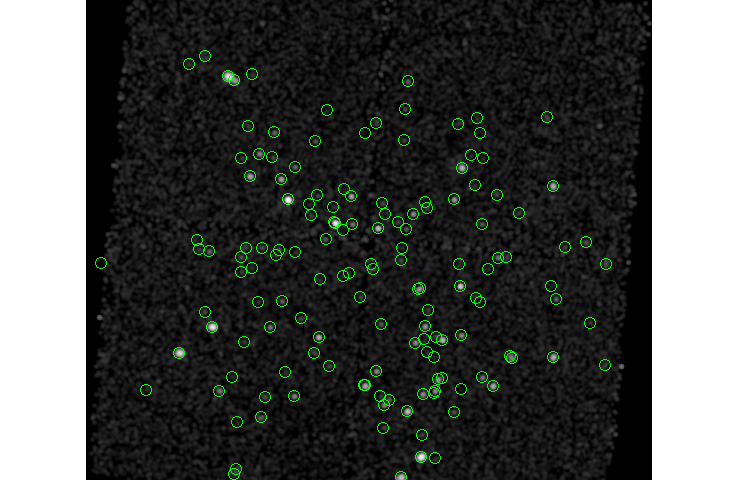### Figure 5: The CSC sources

The circles shown by ds9 are "points", since there is no region information in the TSV file created by search_csc. As you zoom in the circles will get smaller, as can be seen in the inset view, which shows the two close sources in the North-East of the field (it is the same pair as shown in Figure 7).Note

In this case the source list is from Chandra, and so we expect to see a one-to-one correspondance between the source list and the sources seen in the data (e.g. Figure 5). However, there is no reason that an X-ray source list has to be used.

### Creating a source list with wavdetect

For this example we shall use wavdetect to create the source list, but other methods can be used. The image and exposure map, created earlier, are going to be used. For your actual analysis it is likely that you should use a smaller binning factor than the value used here (4), which may mean increasing the set of scales used by wavdetect.

```unix% cd ..
unix% mkdir wav
unix% punlearn wavdetect
unix% pset wavdetect regfile=wav/src.reg
unix% pset wavdetect scales="2 4 8 16"
unix% wavdetect fimg/broad_thresh.img wav/src.fits wav/src.scell wav/src.img wav/src.nbkg mode=h
unix% dmlist wav/src.fits counts
80
```

and the resulting source list is shown in Figure 6.

### Figure 6: Sources detected by wavdetect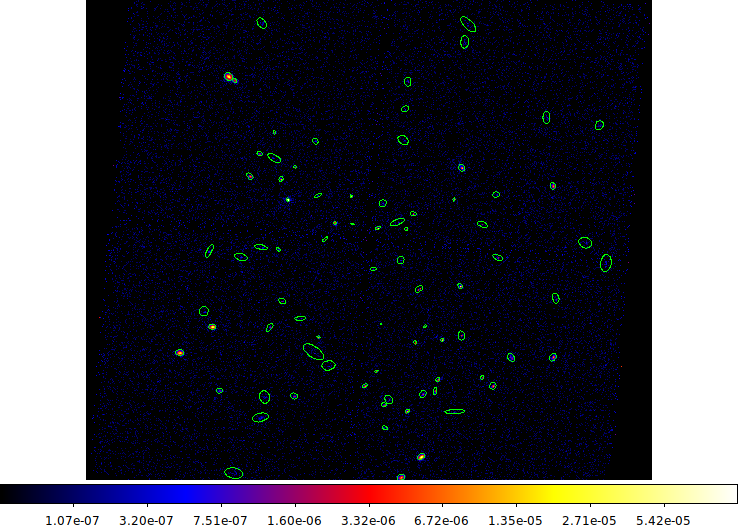### Figure 6: Sources detected by wavdetect

```unix% ds9 fimg/broad_flux.img -region wav/src.fits -log -cmap b
```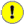Important

Users are reminded that the output from wavdetect is only a list of candidate sources and that the source properties, including the region defintion, are approximations.

Although the source list created by wavdetect can be used as a region file (e.g. Figure 6), it is not usable here because:

1. there are no background regions,

2. and there is no check for overlapping sources.

Both issues can be addresed by using the roi tool. Given a set of regions, it outputs one region per source with a source region in the SRCREG extension and the corresponding background region in the BKGREG extension. The background is an annulus around the source, with a number of parameters - described in ahelp roi - for controlling the size and spacing relative to the source area. The parameters chosen here - namely group=exclude and targetbkg=target - ensure that each source in the input file has a region, that any other overlapping sources are excluded from both the source and background regions, and that there is a single annulus used for the background (rather than multiple annuli, one for each source that overlaps the output source). The roi tool has many capabilities and you are encouraged to change the parameters to see whether they are better for your science needs.

```unix% mkdir sources
unix% punlearn roi
unix% pset roi group=exclude bkgfactor=1
unix% pset roi outsrcfile="sources/src%02d.fits"
unix% pset roi targetbkg=target
unix% roi wav/src.fits "region(repro/acisf06436_repro_fov1.fits)"
Input streak region ():
Output source list (sources/src%02d.fits):
unix% ls -1 sources/src*fits | wc -l
81
unix% dmlist sources/src01.fits blocks

--------------------------------------------------------------------------------
Dataset: sources/src01.fits
--------------------------------------------------------------------------------

Block Name                          Type         Dimensions
--------------------------------------------------------------------------------
Block    1: PRIMARY                        Null
Block    2: SRCREG                         Table         5 cols x 1        rows
Block    3: BKGREG                         Table         5 cols x 2        rows
```Numbering

The outsrcfile parameter uses %02d rather than %d so that later processing, in particular the splitroi call, can easily match up the correct source and background regions; if you have more than 99 sources use a larger number of characters, such as %04d.

In Figure 7 we show the source and background regions created by roi for a source which has a close neighbor.

### Figure 7: A source and background region, created by roi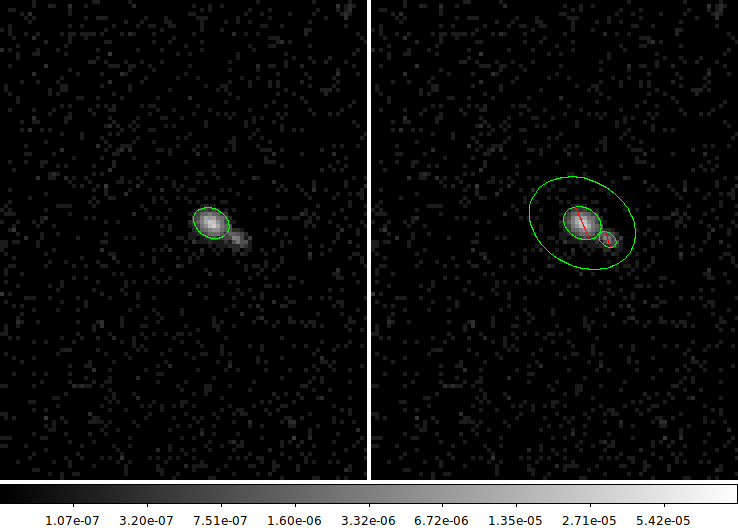### Figure 7: A source and background region, created by roi

Since the source regions are for this source is so close the neighboring source, the background regions created by roi require both included and excluded regions for each background; each source is excluded from each other's background.

```unix% ds9 fimg/broad_flux.img -log -region sources/src73.fits \
-pan to 3460 4780 -zoom 4 fimg/broad_flux.img -log \
-region "sources/src73.fits[bkgreg]" -pan to 3460 4780
```

Note how the extension bkgreg had to be specified for the background, otherwise ds9 assumes you mean the first region block (srcreg in this case).

The final step is to create a list of source and background regions from the output of roi, which can be performed by the splitroi script:

```unix% splitroi sources/src\*fits sources/wav
unix% ls -1 sources/wav*reg
sources/wav.bg.reg
sources/wav.src.reg
```

## Multiple sources

We start by using the CSC results, whereby srcflux is given a list of positions only. We then show how region information can be included by using the wavdetect source list.

### Using a catalog

Here we try running srcflux with just a source list, letting it calculate the source and background regions. In this case we are going to use the source list from the CSC, but data from other wave bands can be used.

To keep the run-time manageable we choose a subset of sources, where row number 403 is the source used in the single-source analysis above. A single nH value is assumed here, even though it does vary across the ACIS field.Multiple sources

The CSC results created by search_csc contain multiple rows with the same source; if these multiple occurrences are not filtered out at this stage then srcflux will treat these repeats as separate sources, resulting in sources with zero areas (due to the areas cancelling out), and then flux=NAN).

```srcflux
infile = repro/acisf06436_repro_evt2.fits
pos = csc2.fits[#row=3,15,18,403,430]
outroot = multiple-csc/run
bands = default
srcreg =
bkgreg =
bkgresp = yes
psfmethod = ideal
psffile =
conf = 0.9
binsize = 1
rmffile =
arffile =
model = xspowerlaw.pow1
paramvals = pow1.phoindex=2
absmodel = xsphabs.abs1
absparams = abs1.nh=0.1464
abund = angr
fovfile =
asolfile =
mskfile =
bpixfile =
dtffile =
ecffile = CALDB
parallel = yes
nproc = INDEF
tmpdir = ./
random_seed = -1
clobber = yes
verbose = 1
mode = ql

Processing OBI 001
Extracting counts
Setting Ideal PSF : alpha=1 , beta=0
Getting net rate and confidence limits
Getting model independent fluxes
Getting model fluxes
Getting photon fluxes
Running tasks in parallel with 4 processors.
Making response files for multiple-csc/run_0001
Making response files for multiple-csc/run_0002
Making response files for multiple-csc/run_0003
Making response files for multiple-csc/run_0004
Running modeflux for region 2
Making response files for multiple-csc/run_0005
Running modeflux for region 1
Running modeflux for region 4
Running modeflux for region 3
Appending flux results onto output
Appending photflux results onto output
Computing Net fluxes
Scaling model flux confidence limits

Summary of source fluxes

Position                               0.5 - 7.0 keV
Value        90% Conf Interval
#0001|3 28 28.81 +31 20 36.9  Rate           0.000314 c/s (0.000155,0.000523)
Flux           6.96E-15 erg/cm2/s (3.44E-15,1.16E-14)
Mod.Flux       5.84E-15 erg/cm2/s (2.89E-15,9.71E-15)
Unabs Mod.Flux 7.17E-15 erg/cm2/s (3.55E-15,1.19E-14)

#0002|3 28 36.50 +31 19 28.7  Rate           0.000585 c/s (0.000388,0.000834)
Flux           4.12E-15 erg/cm2/s (2.73E-15,5.88E-15)
Mod.Flux       6.87E-15 erg/cm2/s (4.55E-15,9.79E-15)
Unabs Mod.Flux 8.44E-15 erg/cm2/s (5.59E-15,1.2E-14)

#0003|3 28 36.90 +31 17 35.0  Rate           0.00705 c/s (0.00633,0.00779)
Flux           6.31E-14 erg/cm2/s (5.66E-14,6.97E-14)
Mod.Flux       8.29E-14 erg/cm2/s (7.44E-14,9.15E-14)
Unabs Mod.Flux 1.02E-13 erg/cm2/s (9.14E-14,1.12E-13)

#0004|3 29 29.26 +31 18 34.6  Rate           0.0391 c/s (0.0374,0.0408)
Flux           4.98E-13 erg/cm2/s (4.76E-13,5.19E-13)
Mod.Flux       4.36E-13 erg/cm2/s (4.17E-13,4.56E-13)
Unabs Mod.Flux 5.36E-13 erg/cm2/s (5.13E-13,5.6E-13)

#0005|3 29 46.29 +31 20 39.3  Rate           0 c/s (NAN,7.83E-05)
Outside FOV                  Flux           NAN erg/cm2/s (NAN,NAN)
Mod.Flux       NAN erg/cm2/s (NAN,NAN)
Unabs Mod.Flux NAN erg/cm2/s (NAN,NAN)
```

Note that the center of the last region (row number 430) lies outside the FOV, even though it overlaps the chip slightly (Figure 8).

### Figure 8: Source at the edge of the chip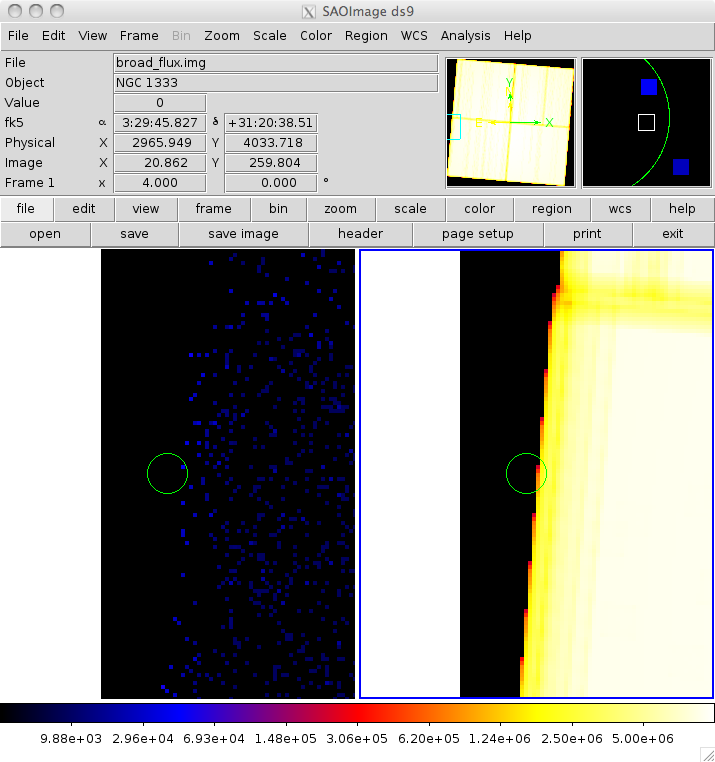### Figure 8: Source at the edge of the chip

```unix% ds9 fimg/broad_flux.img -log -cmap b fimg/broad_thresh.expmap \
-pan to 3:29:46 31:20:39 wcs fk5 -zoom 4 -match frame wcs \
```

The left frame shows the fluxed image and the right frame the broad-band exposure map. Here we zoom in on the last point, showing that the source region just intersects the observation.

Note that we are able to use the output of srcflux as a region file.

Since we have multiple sources, the output file contains many rows, unlike in the single-source case:

```unix% dmlist multiple-csc/run_broad.flux"[cols near_chip_edge,inside_fov,upper_limit]" data,clean
#  NEAR_CHIP_EDGE INSIDE_FOV UPPER_LIMIT
TRUE   TRUE   FALSE
FALSE  TRUE   FALSE
FALSE  TRUE   FALSE
FALSE  TRUE   FALSE
TRUE   FALSE  TRUE
#  COMPONENT  PSFFRAC              BG_PSFFRAC
1                  1.0                    0
2                  1.0                    0
3                  1.0                    0
4                  1.0                    0
5                  1.0                    0
```

The PSF correction is 1/0 for all the sources because the psfmethod parameter was set to ideal. The COMPONENT value refers to the numbering by srcflux and not the row numbers from the original file.

The count rate and fluxes are:

```unix% dmlist "multiple-csc/run_broad.flux[cols net_rate_aper,net_flux_aper,net_mflux_aper,net_umflux_aper]" data,clean
#  NET_RATE_APER        NET_FLUX_APER        NET_MFLUX_APER       NET_UMFLUX_APER
0.000314272      6.958363555E-15      5.836973856E-15      7.171372768E-15
0.000585384      4.123242354E-15      6.870066624E-15      8.440651896E-15
0.007054080        6.3107065E-14       8.29207104E-14      1.018750234E-13
0.0390620      4.975631551E-13       4.36439726E-13       5.36204074E-13
0                  NaN                  NaN                  NaN
```
```unix% dmlist "multiple-csc/run_broad.flux[cols net_mflux_aper,net_mflux_aper_lo,net_mflux_aper_hi]" data,clean
#  NET_RATE_APER        NET_RATE_APER_LO     NET_RATE_APER_HI
0.000314272          0.000155482          0.000522684
0.000585384          0.000387565          0.000834341
0.007054080          0.006326250          0.007786340
0.0390620           0.03736030           0.04077410
0                  NaN          7.83079E-05
```
```unix% dmlist "multiple-csc/run_broad.flux[cols net_umflux_aper,net_umflux_aper_lo,net_umflux_aper_hi]" data,clean
#  NET_UMFLUX_APER      NET_UMFLUX_APER_LO   NET_UMFLUX_APER_HI
7.171372768E-15      3.547943758E-15       1.19271262E-14
8.440651896E-15      5.588299735E-15      1.203036288E-14
1.018750234E-13       9.13637025E-14      1.124503223E-13
5.36204074E-13      5.128448381E-13      5.597060707E-13
NaN                  NaN                  NaN
```

Comparing the results for source number 403 with those from above (picking the same choice of spectral model and aperture correction strategies), we see similar results:

```unix% dmlist "multiple-csc/run_broad.flux[component=4][cols x,y,r,net_rate_aper,net_rate_aper_lo,net_rate_aper_hi]" data,clean
#  sky(X,Y)                                 R                                     NET_RATE_APER        NET_RATE_APER_LO     NET_RATE_APER_HI
3423.410      3796.0            9.630440                    0            0.0390620           0.03736030           0.04077410
```

### Using source and region files

In this example a source list and a set of source and background regions are given. Since there are 81 sources this can take a long time (note that srcflux will use all available CPUs on your machine for parts of the analysis; this can be controlled with the parallel and nproc parameters).

```unix% punlearn srcflux
unix% pset srcflux model=xspowerlaw.pow1 paramvals="pow1.phoindex=2"
unix% pset srcflux absmodel=xsphabs.abs1 absparams="abs1.nh=0.1464"
unix% pset srcflux psfmethod=arfcorr
unix% pset srcflux srcreg=@-sources/wav.src.reg
unix% pset srcflux bkgreg=@-sources/wav.bg.reg
```Stacks and directory names

The stacks for the source and background regions use the "@-" syntax rather than just "@ to avoid the directory name containing the stack file being appended to each region, as shown in the first stk_read_num call below:

```unix% stk_read_num @sources/wav.src.reg 1 echo+
sources/Ellipse(4151.58,3178.88,16.4402,11.3052,27.7724)
Ellipse(4151.58,3178.88,16.4402,11.3052,27.7724)
```

See the stack ahelp page for more information on stack handling in CIAO tools.

```unix% srcflux repro/acisf06436_repro_evt2.fits wav/src.fits multiple-wav/run
srcflux
infile = repro/acisf06436_repro_evt2.fits
pos = wav/src.fits
outroot = multiple-wav/run
bands = default
srcreg = @-sources/wav.src.reg
bkgreg = @-sources/wav.bg.reg
bkgresp = yes
psfmethod = arfcorr
psffile =
conf = 0.9
binsize = 1
rmffile =
arffile =
model = xspowerlaw.pow1
paramvals = pow1.phoindex=2
absmodel = xsphabs.abs1
absparams = abs1.nh=0.1464
abund = angr
fovfile =
asolfile =
mskfile =
bpixfile =
dtffile =
ecffile = CALDB
parallel = yes
nproc = INDEF
tmpdir = ./
random_seed = -1
clobber = yes
verbose = 1
mode = h

Processing OBI 001
Extracting counts
Making PSF models
Getting PSF fraction by running arfcorr 1
Getting PSF fraction by running arfcorr 2
Getting PSF fraction by running arfcorr 3
Getting PSF fraction by running arfcorr 4
Getting PSF fraction by running arfcorr 5
...
Getting PSF fraction by running arfcorr 80
Combining PSF fractions together
Getting net rate and confidence limits
Getting model independent fluxes
Getting model fluxes
Getting photon fluxes
Running tasks in parallel with 4 processors.
...
Running modeflux for region 79
Running modeflux for region 80
Appending flux results onto output
Appending photflux results onto output
Computing Net fluxes
Scaling model flux confidence limits

Summary of source fluxes

Position                               0.5 - 7.0 keV
Value        90% Conf Interval
#0001|3 29 0.29 +31 13 38.4   Rate           0.0183 c/s (0.017,0.0196)
Flux           1.97E-13 erg/cm2/s (1.83E-13,2.11E-13)
Mod.Flux       2.12E-13 erg/cm2/s (1.97E-13,2.27E-13)
Unabs Mod.Flux 2.61E-13 erg/cm2/s (2.42E-13,2.79E-13)

#0002|3 28 57.20 +31 14 18.8  Rate           0.0626 c/s (0.0602,0.0649)
Flux           5.52E-13 erg/cm2/s (5.31E-13,5.73E-13)
Mod.Flux       7.38E-13 erg/cm2/s (7.1E-13,7.66E-13)
Unabs Mod.Flux 9.06E-13 erg/cm2/s (8.73E-13,9.41E-13)

#0003|3 29 2.77 +31 15 15.8   Rate           0.000385 c/s (0.000206,0.000627)
Flux           9.29E-15 erg/cm2/s (4.96E-15,1.51E-14)
Mod.Flux       4.11E-15 erg/cm2/s (2.2E-15,6.69E-15)
Unabs Mod.Flux 5.05E-15 erg/cm2/s (2.7E-15,8.22E-15)

#0004|3 29 21.85 +31 15 35.9  Rate           0.0011 c/s (0.000792,0.00147)
Flux           2.69E-14 erg/cm2/s (1.93E-14,3.58E-14)
Mod.Flux       1.2E-14 erg/cm2/s (8.59E-15,1.59E-14)
Unabs Mod.Flux 1.47E-14 erg/cm2/s (1.06E-14,1.96E-14)
...
#0079|3 28 49.93 +31 28 29.6  Rate           0.000407 c/s (0.000166,0.000695)
Flux           6.43E-15 erg/cm2/s (2.62E-15,1.1E-14)
Mod.Flux       4.57E-15 erg/cm2/s (1.86E-15,7.8E-15)
Unabs Mod.Flux 5.62E-15 erg/cm2/s (2.29E-15,9.58E-15)

#0080|3 28 28.81 +31 20 39.5  Rate           0.000314 c/s (8.66E-05,0.000589)
Flux           3.86E-15 erg/cm2/s (1.07E-15,7.25E-15)
Mod.Flux       6E-15 erg/cm2/s (1.66E-15,1.13E-14)
Unabs Mod.Flux 7.37E-15 erg/cm2/s (2.03E-15,1.38E-14)
```

The screen output will indicate whether there were issues with any source, but we can also look at the INSIDE_FOV and UPPER_LIMIT columns to find problem cases; in this case there aren't any.

```unix% dmlist "multiple-wav/run_broad.flux[inside_fov=false||upper_limit=true]" counts
0
```

We can use matplotlib to visualize the results; for example Figure 9 was created with the following:

```unix% python

>>> import matplotlib.pylab as plt
>>>
>>> cpt = cr.get_column("COMPONENT").values
>>> umflux = cr.get_column("NET_UMFLUX_APER").values
>>> umflux_units = cr.get_column("NET_UMFLUX_APER").unit
>>> umflux_lo = cr.get_column("NET_UMFLUX_APER_LO").values
>>> umflux_hi = cr.get_column("NET_UMFLUX_APER_HI").values
>>> theta = cr.get_column("THETA").values
>>> theta_units = cr.get_column("THETA").unit
>>> ef_lo = umflux-umflux_lo
>>> ef_hi = umflux_hi-umflux
>>>
>>> plt.subplots(2, 1, sharex='col', figsize=(8,6))
>>> plt.subplot(2,1,1)
>>> plt.errorbar(cpt,umflux,yerr=[ef_lo,ef_hi],linestyle="none",marker="o", markersize=2)
>>> plt.yscale("log")
>>> plt.ylabel("Unabsorbed flux ({})".format(umflux_units))
>>> plt.title("srcflux output for obsid 6436")
>>>
>>> plt.subplot(2,1,2)
>>> plt.plot(cpt,theta, linestyle="none", marker="x")
>>> plt.ylabel("Theta ({})".format(theta_units))
>>> plt.xlabel("Source number")
```

### Figure 9: Unabsorded fluxes for the sources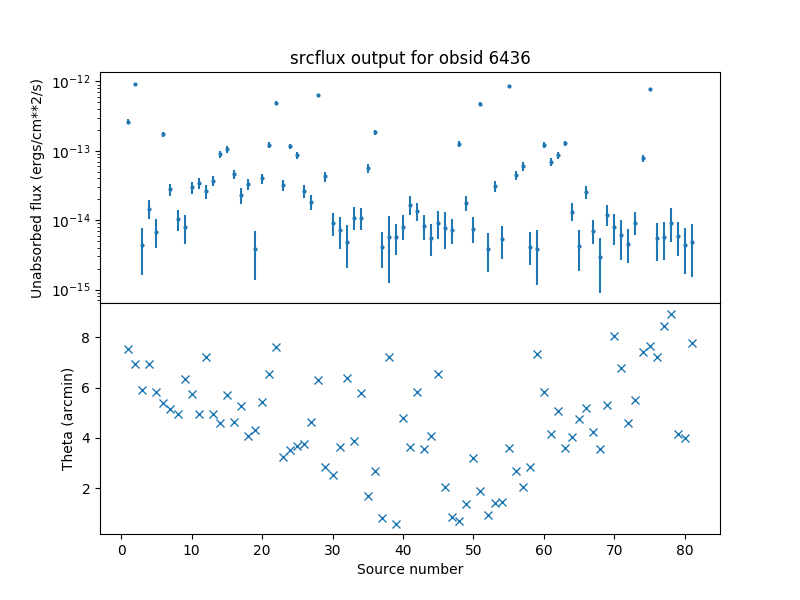## Something went wrong

Here's a few things that can go wrong:

### Coordinate issues

The error

```# srcflux (16 Oct 2013): ERROR An error occurred while running 'psfsize_srcs':
psfsize_srcs
infile = repro/acisf06436_repro_evt2.fits
pos = 52.3235594436 31.3791611941
outfile = test_srcs.fits
energy = 1.0
ecf = 0.9
psffile = CALDB
verbose = 1
clobber = no
mode = hl

# psfsize_srcs (2 Oct Sep 2013): ERROR pos value must be a filename or have a +, -, or comma separator
```

means that the pos parameter is invalid; in this particular case srcflux was run with pos="52.3235594436 31.3791611941" which is missing the sign on the declination; it should have been pos="52.3235594436 +31.3791611941".

This error is also seen when the pos argument is given a file that does not exist or can not be opened.

### Sources at the edge of the field of view

You can see this error, when using psfmethod=quick:

```# src_psffrac (5 Aug 2013): ERROR Only circle shapes are allowed
```

which means that your source region (or one of them) intersects the FOV or there's another source overlapping it that gets cut out. In this case try psfmethod=quick.

### No counts

The following message means that the source region contains no counts. In this case an upper limit should be calculated but currently is not (the upper limit will be set to NaN instead).

```An error occurred while running 'fluximage':
Running fluximage
Version: 13 December 2013

# fluximage (13 December 2013): ERROR There are no events in repro/acisf06436_repro_evt2.fits...
```

## History

 12 Dec 2013 New for CIAO 4.6. 31 Dec 2013 Added note about wavdetect source candidates. 04 Dec 2014 Review for CIAO 4.7. Updated dmcoords call as the aspect solution file is no longer required; the information needed to account for the SIM drift is now included in the headers. 10 Dec 2018 Updated for CIAO 4.11. fluximage now produces the psfmap. 02 Apr 2019 Updated to use matplotlib for plotting. 28 Jan 2021 Updated to use csc2 sources. Updated all examples for latest calibrations and due to changes in sky(x,y) coordinates. 23 Feb 2022 Review for CIAO 4.14. Updated for Repro5/CALDB 4.9.6.